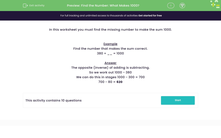# Use Inverse Operation to Total 1,000

In this worksheet, students will use subtraction to find the missing number to make the total 1,000.Key stage:  KS 2

Curriculum topic:   Number: Addition and Subtraction

Curriculum subtopic:   Use Correct Methods to Problem Solve

Difficulty level:#### Worksheet Overview

In this activity, we will be finding the missing number to make the total of 1,000.

Example

Find the number that makes the calculation correct.

380 + __ =  1,000

The opposite (inverse) of adding is subtracting.

So we work out 1,000 - 380

We can do this in stages 1,000 - 300 = 700

700 - 80 = 620

Does that make sense?

Let's practise this in the following questions.### What is EdPlace?

We're your National Curriculum aligned online education content provider helping each child succeed in English, maths and science from year 1 to GCSE. With an EdPlace account you’ll be able to track and measure progress, helping each child achieve their best. We build confidence and attainment by personalising each child’s learning at a level that suits them.

Get started